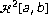# Smooth fractal interpolation

## Abstract

Fractal methodology provides a general frame for the understanding of real-world phenomena. In particular, the classical methods of real-data interpolation can be generalized by means of fractal techniques. In this paper, we describe a procedure for the construction of smooth fractal functions, with the help of Hermite osculatory polynomials. As a consequence of the process, we generalize any smooth interpolant by means of a family of fractal functions. In particular, the elements of the class can be defined so that the smoothness of the original is preserved. Under some hypotheses, bounds of the interpolation error for function and derivatives are obtained. A set of interpolating mappings associated to a cubic spline is defined and the density of fractal cubic splines inis proven.

## References

1. Ahlberg JH, Nilson EN, Walsh JL: The Theory of Splines and Their Applications. Academic Press, New York; 1967:xi+284.

2. Barnsley MF: Fractal functions and interpolation. Constructive Approximation 1986,2(4):303–329.

3. Barnsley MF: Fractals Everywhere. Academic Press, Massachusetts; 1988:xii+396.

4. Barnsley MF, Harrington AN: The calculus of fractal interpolation functions. Journal of Approximation Theory 1989,57(1):14–34. 10.1016/0021-9045(89)90080-4

5. Chand AKB, Kapoor GP: Generalized cubic spline fractal interpolation functions. SIAM Journal on Numerical Analysis 2006,44(2):655–676. 10.1137/040611070

6. Ciarlet PG, Schultz MH, Varga RS: Numerical methods of high-order accuracy for nonlinear boundary value problems. I. One dimensional problem. Numerische Mathematik 1967,9(5):394–430. 10.1007/BF02162155

7. Hall CA, Meyer WW: Optimal error bounds for cubic spline interpolation. Journal of Approximation Theory 1976,16(2):105–122. 10.1016/0021-9045(76)90040-X

8. Hutchinson JE: Fractals and self-similarity. Indiana University Mathematics Journal 1981,30(5):713–747. 10.1512/iumj.1981.30.30055

9. Laurent P-J: Approximation et optimisation. Hermann, Paris; 1972:xiii+531.

10. Navascués MA, Sebastián MV: Some results of convergence of cubic spline fractal interpolation functions. Fractals 2003,11(1):1–7. 10.1142/S0218348X03001550

11. Navascués MA, Sebastián MV: Fitting curves by fractal interpolation: an application to the quantification of cognitive brain processes. In Thinking in Patterns: Fractals and Related Phenomena in Nature. Edited by: Novak MM. World Scientific, New Jersey; 2004:143–154.

12. Navascués MA, Sebastián MV: Generalization of Hermite functions by fractal interpolation. Journal of Approximation Theory 2004,131(1):19–29. 10.1016/j.jat.2004.09.001

13. Navascués MA, Sebastián MV: A fractal version of Schultz's theorem. Mathematical Inequalities & Applications 2005,8(1):63–70.

14. Stoer J, Bulirsch R: Introduction to Numerical Analysis. Springer, New York; 1980:ix+609.

## Author information

Authors

### Corresponding author

Correspondence to M. A. Navascués.

## Rights and permissions

Reprints and Permissions

Navascués, M.A., Sebastián, M.V. Smooth fractal interpolation. J Inequal Appl 2006, 78734 (2006). https://doi.org/10.1155/JIA/2006/78734

• Revised:

• Accepted:

• Published:

• DOI: https://doi.org/10.1155/JIA/2006/78734

### Keywords

• Classical Method
• Interpolation Error
• Fractal Function
• General Frame
• Fractal Technique